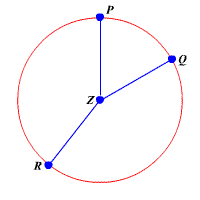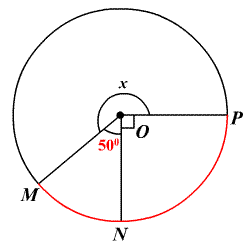# Central Angles

A central angle is an angle with its vertex at the center of a circle, with its sides containing two radii of the circle.In the figure above, $\angle PZQ,\angle QZR$ , and $\angle RZP$ are central angles.

Sum of Central Angles: The sum of the measures of the central angles of a circle with no points in common is $360°$ .

For example, in the figure above,

$m\angle PZR+m\angle RZQ+m\angle QZP=360°$ .

Example:

Find the value of $x$ .The sum of the measures of the central angles of a circle with no interior points in common is $360°$ . So,

$\begin{array}{l}m\angle MON+m\angle NOP+m\angle POM=360°\\ 50°+90°+x=360°\end{array}$

Simplify.

$140°+x=360°$

Subtract $140°$ from each side.

$x=220°$